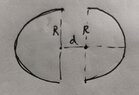# Finding the capacitance of two separated hemispheres

jiajie
New poster has been reminded to show their work on schoolwork problems
Homework Statement:
an interesting problem to discuss
Relevant Equations:
How to calculate a pair of adjacent hemispheres capacitance?
like the picture, two adjacent hemispheres（radius R, distance d, assume the charge is ±Q of each side（assume evenly distributed）, can we calculate its capacitance?

#### Attachments

•IMG_20210516_212925_edit_27864824671788.jpg
37.2 KB · Views: 58
Last edited:

Staff Emeritus
Homework Helper
Gold Member
What is the definition of capacitance?

Gordianus
The assumption of uniformly distributed charge doesn't lead to equipotential hemispheres. In this case, calculating the capacitance isn't an easy task.

Keith_McClary
The assumption of uniformly distributed charge doesn't lead to equipotential hemispheres. In this case, calculating the capacitance isn't an easy task.
They can't be conductors, so I don't think there is any standard definition of capacitance.

jiajie
can we assume the electrical field is horizontally paralleled to simply it

Gordianus
can we assume the electrical field is horizontally paralleled to simply it
Unfortunately, it isn't that simple. I checked whether Smythe had addressed this case but I had no luck.